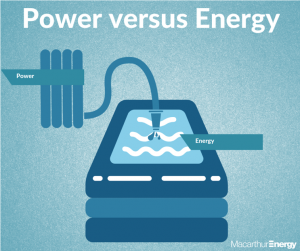Did you know there is a difference between solar power and solar energy? Don’t worry, I bet many solar reps don’t either. In this blog, I will explain the difference between the two and why it’s so important to understand these two terms when learning the fundamentals of a solar system.

Power = Electricity (kW)
Energy = Amount of electricity used (kWh)

First let’s break down the abbreviations you may have heard of:
k – meaning kilo or one thousand
W – meaning watt
h – meaning hour
kW – The measurement for power is kilowatts
kWh – The measurement for energy is kilowatt hours

Now the easiest way to explain the difference between solar power and solar energy is to imagine you are filling up a pool with water from the hose.
The water, flowing through the hose represents power. All the water collected in the pool, is referred to as energy.You might be thinking to yourself yeah, yeah who cares, but the reason this is so important when considering investing in solar, is because it gives you a greater understanding of the size of the system you may require for your needs.

For instance, I once had a customer tell me they need a 10kW solar system. They made this assumption based on their energy usage figure written on their electricity bill which stated they used 10kWh per day. For me to install a 10kW solar system, this would come at a cost to the customer of \$10-15k and would consist of 30-35 solar panels.
But in this case, the customer has assumed that the 10kWh he was using would equate to a 10kW solar system. This is not correct.
A 10kW solar system would be far too large for his needs. In reality, a 2-3kW solar system or 6-10 solar panels would be more than enough to suit his household needs and only come at a cost of \$3-5k.

Now let’s put it into perspective. A solar system may consist of 10x 300w solar panels and it would be commonly referred to as a 3kW system. This is what we call the solar peak power rating.
The most common mistake people make when investing in researching solar systems is assuming that a 3kW system will produce 3kW’s of power whenever the sun is shining. This is just not true.
A 3kW system will rarely, if ever, produce this much power. The peak power rating would only occur if all conditions were perfect, this includes the temperature, the angle of the solar panels in respect to true North, the height of the sun and the efficiency of the solar panels and inverter.

On average for the Sydney and Macarthur area, we estimate a 3kw Solar power system will produce 2.4kW of power for five hours a day giving you approximately 12kWh per day.
2.4kW x 5hours = 12kWh

The rule of thumb for a quick calculation of a solar power systems output in Sydney or Macarthur is 80% of the peak power rating multiplied by five hours per day. This will give you the average daily energy generation of the system.